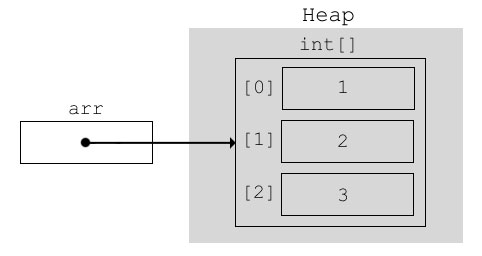# Difference Between Object and Reference in Java

Sometimes it gets confusing for people who are new to java as to what is an Object and what is a Reference. Knowing the difference between them is critical in understanding a lot of key concepts of java.

Lets start with an example. You must have contacts of many people in your mobile. You can use a contact to call a person. The person is actually somewhere else, but the contact helps you to call them. You can think of a Reference variable to be a contact and Object to be the actual person you are calling. Basically a reference variable just points to an actual object. For example if we have a class,

class Person {
String name = "Eric";
int age = 25;
}

If you instantiate the class Person like this

Person p = new Person();

Here p is a reference variable which points to an object of class Person. The actual object of class Person created in that statement resides on the heap. The reference variable p only contains the memory address of Person class object on the heap. So the actual value of p can be said to be an address on the heap.Now if you notice, the String name in class Person is itself a reference variable. So it will point to a String object on the heap. So the person object that we created above, can be represented by the following diagram,Here the reference variable p points to an object of type Person. The name reference variable in Person object points to String object on the heap.

Now that we know what reference and object is, we will also cover final reference variables here. If you create a reference variable like this,

final Person p = new Person();

Here the reference variable p is final. What this means is that now we cannot change its value. The value of reference variable p is the address of the Person object on the heap. So we cannot modify that. But we can surely modify the state of the object. So I can change the name and/or age of the Person object referenced by p. So this code is valid,

final Person p = new Person();
p.name = "Bill";
p.age = 35;

Here we are changing the state of the object referenced by p. This is allowed as we are not changing the value of the reference variable p itself. If I try something like the following, I’ll get a compile time error,

final Person p = new Person();
p = null;  //error, p is final

I can’t change the value of p itself as its final so I get a compile time error if I try to do so.

## Array References

Now that we’ve seen how object references work, lets see how array references work. An array in itself is a reference variable. It points to a group of elements on the heap. If we have an array of primitive data type, then the elements in the array store their actual value. So if we have an int array like the following,

int[] arr = new int[]{1,2,3};

then it can be represented via a diagram as follows,For arrays of non-primitive data types, each element in the array is itself a reference variable (or just reference). So if we have a String array like this,

String[] arr = new String[]{"what","is","this?"};

Then it can be diagrammatically represented as,So basically arr points to an array which has 3 references of type String each pointing to a String in the heap.